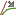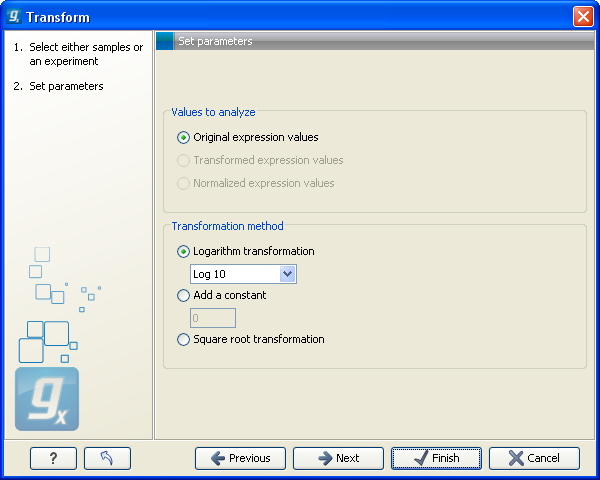## Transformation

The CLC Genomics Workbench lets you transform expression values based on logarithm and adding a constant:

Toolbox | Transcriptomics Analysis ()| Transformation and Normalization | Transform ()

Select a number of samples ( () or ()) or an experiment () and click Next.

This will display a dialog as shown in figure 26.75.Figure 26.75: Transforming expression values.

At the top, you can select which values to transform (see Selecting transformed and normalized values for analysis).

Next, you can choose three kinds of transformation:

• Logarithm transformation. Transformed expression values will be calculated by taking the logarithm (of the specified type) of the values you have chosen to transform.
• 10.
• 2.
• Natural logarithm.
• Adding a constant. Transformed expression values will be calculated by adding the specified constant to the values you have chosen to transform.
• Square root transformation.

Click Next if you wish to adjust how to handle the results. If not, click Finish.